In :
%load_ext watermark

In :
watermark -d -v -a "Sebastian Raschka"

Sebastian Raschka 09/09/2014

CPython 3.4.1
IPython 2.2.0


[More information](https://github.com/rasbt/watermark) about the watermark magic command extension.

# Rejection Sampling¶

While I was listening to the recent episode of the Programming Throwdown podcast on my way home, I heard about this interesting concept of rejection sampling. This is one of those simple ideas combined with the powerful principles of statistics that I find quite fascinating.

At its core, rejection sampling is similar to the popular Monte Carlo sampling with the difference of an additional bound. The goal of rejection sampling is to simplify the task of drawing random samples from a complex probability distribution by using a uniform distribution instead; random samples drawn from the uniform distribution that lie outside certain boundary criteria are rejected, and all samples within the boundary are accepted, respectively.

A mathematical proof can be found here.

Let's use a simple example to illustrate this concept: Our task is to draw random samples from a geometrically-bounded distribution in form a circle in a cartesian coordinate system with a radius of 2 centered at the coordinates x=4 and y=4.

In :
%matplotlib inline
import matplotlib.pyplot as plt

In :
center = (4,4)

""" Function to plot a circle. """
fig = plt.figure(figsize=(6,6))
circle = plt.Circle(center, radius, fill=False, color='b')
plt.ylim([0,8])
plt.xlim([0,8])
fgca = fig.gca()
return fgca

plt.show()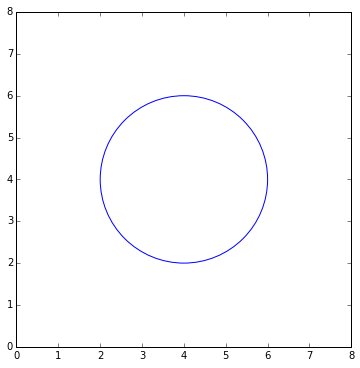Now, we can draw a simple square around the circle that will represent our uniform distribution.

In :
from matplotlib.patches import Rectangle

""" Function to plot a square. """
return fgca

plt.show()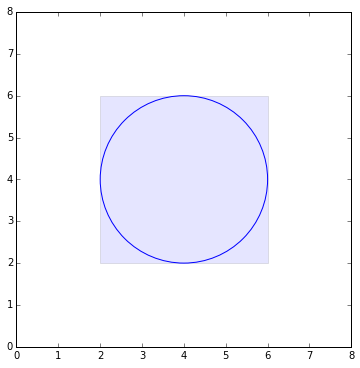Next, we will define a function that generates pseudo-random X and Y coordinates that fall inside the square.

In :
import random
random.seed(567)

In :
def gen_points(n, center, radius):
"""
Function that generates
n x,y coordinates in a square of length radius*2.

"""
x_coords = []
y_coords = []
for i in range(n):
return x_coords, y_coords

In :
x, y = gen_points(1, center, radius)
fgca.plot(x, y, linestyle="", marker="x", color="red")
plt.show()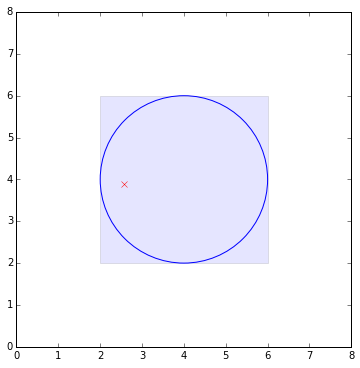Let us generate 1000 random points and check if our function works correctly.

In :
x, y = gen_points(1000, center, radius)
fgca.plot(x, y, linestyle="", marker="x", color="red")
plt.show()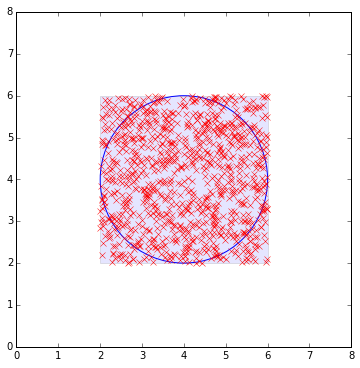The plot above looks fine. In the last step, we only need to reject those points lie outside the circle, which is pretty straight forward using a Euclidean distance measure, that is

\begin{equation} \sqrt{(x-y)^2} = |x-y|. \end{equation}

In :
def reject(radius, center, x_coords, y_coords):
""" Returns those coordinates that fall within the circle. """
x_clean = []
y_clean = []
for x,y in zip(x_coords, y_coords):
if ((x - center)**2 + (y-center)**2)**0.5 <= radius:
x_clean.append(x)
y_clean.append(y)
return x_clean, y_clean


Again, let us do a quick visual check if we correctly removed all points that didn't satisfy the condition $|x-y| \le \text{radius}$.

In :
x_clean, y_clean = reject(radius, center, x, y)
fgca.plot(x_clean, y_clean, linestyle="", marker="x", color="red")
plt.show()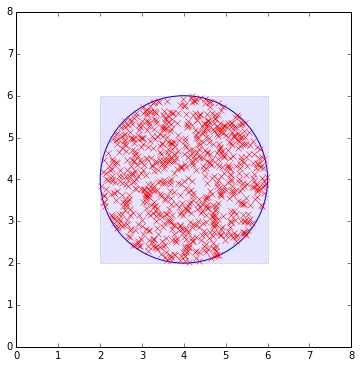Okay, it seems that we successfully wrote some code that can generate pseudo-random samples that fall inside a geometric circle. If this isn't exciting enough, let us use this concept to estimate the area of this circle pretending that we don't know π (pi).

\begin{equation} A_{\text{rectangle}} = (2 \times R)^2 \end{equation}

\begin{equation} \hat{A}_{\text{est_circle}} = A_{\text{rectangle}} \times \frac{\text{# points inside circle}}{\text{# all points}} \end{equation}
In :
def estimate_circle_area(n, center, radius):
""" Returns the estimated circle area via rejection sampling. """
x, y = gen_points(n, center, radius)
x_clean, y_clean = reject(radius, center, x, y)
est_circle_area = rect_area * (len(x_clean)/len(x))

return est_circle_area

In :
print('Estimated circle area: %s' %estimate_circle_area(100000, center, radius))

Estimated circle area: 12.56224


Now, let's double-check how close we got using the more accurate equation:

\begin{equation} A_{\text{circle}} = \pi \times R^2 \end{equation}
In :
from math import pi

print('Circle area using pi: %s' %(pi*radius**2))

Circle area using pi: 12.566370614359172


Who would have guessed what comes next: Let us use our rejection sampling method to estimate pi itself:

\begin{equation} \hat{\pi} = \frac{\hat{A}_{\text{est_circle}}}{R^2} \end{equation}

In :
def approximate_pi(n, center, radius):
""" Returns an approximation of pi via rejection sampling. """

Pi estimate: 1.6 (n=10)